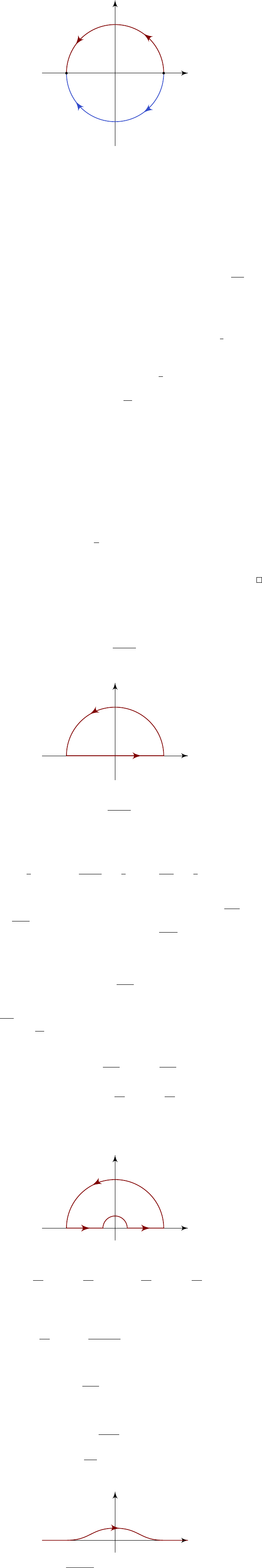4The calculus of residues

IB Complex Methods4.4 Jordan’s lemma
So far, we have bounded the semi-circular integral in a rather crude way, with
Z
γ
R
f(z)e
iλz
dz
R sup
|z|=R
|f(z)|.
This works for most cases. However, sometimes we need a more subtle argument.
Lemma
(Jordan’s lemma)
.
Suppose that
f
is an analytic function, except for a
finite number of singularities, and that f(z) 0 as |z| .
γ
R
γ
0
R
R R
×
×
×
Then for any real constant λ > 0, we have
Z
γ
R
f(z)e
iλz
dz 0
as R , where γ
R
is the semicircle of radius R in the upper half-plane.
For
λ <
0, the same conclusion holds for the semicircular
γ
0
R
in the lower
half-plane.
Such integrals arise frequently in Fourier transforms, as we shall see in the
next chapter.
How can we prove this result?
The result is easy to show if in fact
f
(
z
) =
o
(
|z|
1
) as
|z|
, i.e.
f(z)
|z|
0
as |z| , since |e
iλz
| = e
λ Im z
1 on γ
R
. So
Z
γ
R
f(z)e
iλz
dz
πR · o(R
1
) = o(1) 0.
But for functions decaying less rapidly than
o
(
|z|
1
) (e.g. if
f
(
z
) =
1
z
), we need
to prove Jordan’s lemma, which extends the result to any function
f
(
z
) that
tends to zero at infinity.
Proof. The proof relies on the fact that for θ
0,
π
2
, we have
sin θ
2θ
π
.
So we get
Z
γ
R
f(z)e
iλz
dz
=
Z
π
0
f(Re
)e
iλRe
iRe
dθ
R
Z
π
0
|f(Re
)|
e
iλRe
dθ
2R sup
zγ
R
|f(z)|
Z
π/2
0
e
λR sin θ
dθ
2R sup
zγ
R
|f(z)|
Z
π/2
0
e
2λRθ
dθ
=
π
λ
(1 e
λR
) sup
zγ
R
|f(z)|
0,
as required. Same for γ
0
R
when λ < 0.
Note that for most cases, we don’t actually need it, but can just bound it by
R sup
|z|=R
f(z).
Example. Suppose we want to compute
I =
Z
0
cos αx
1 + x
2
dx,
where α is a positive real constant. We consider the following contour:
γ
0
γ
R
R R
×
i
and we compute
Re
Z
γ
e
iαz
1 + z
2
dz.
Along
γ
0
, we obtain 2
I
as
R
. On
γ
R
, we do not actually need Jordan’s
lemma to show that we obtain zero in the limit, but we can still use it to save
ink. So
I =
1
2
Re
2πi res
z=i
e
iαz
1 + z
2
=
1
2
Re
2πi
e
α
2i
=
1
2
πe
α
.
Note that taking the real part does not do anything here the result of the
integral is completely real. This is since the imaginary part of
R
γ
e
iαz
1+z
2
d
z
is
integrating
sin αz
1+z
2
, which is odd and vanishes.
Note that if we had attempted to integrate
R
γ
cos αz
1+z
2
d
z
directly, we would
have found that
R
γ
R
6→ 0. In fact, cos αz is unbounded at .
Example. We want to find
I =
Z
−∞
sin x
x
dx.
This time, we do require Jordan’s lemma. Here we have an extra complication
while
sin x
x
is well-behaved at the origin, to perform the contour integral, we
need to integrate
e
iz
z
instead, which is singular at the origin.
Instead, we split the integral in half, and write
Z
−∞
= lim
ε0
R→∞
Z
ε
R
sin x
x
dx +
Z
R
ε
sin x
x
dx
!
= Im lim
ε0
R→∞
Z
ε
R
e
iz
x
dx +
Z
R
ε
e
iz
x
dx
!
We now let
C
be the contour from
R
to
ε
, then round a semi-circle
C
ε
to
ε
,
then to
R
, then returning via a semi-circle
C
R
R
. Then
C
misses all
our singularities.
R
ε
C
ε
ε
R
C
R
×
Since C misses all singularities, we must have
Z
ε
R
e
iz
z
dz +
Z
R
ε
e
iz
z
dz =
Z
C
ε
e
iz
z
dz
Z
C
R
e
iz
z
dz.
By Jordan’s lemma, the integral around
C
R
vanishes as
R
. On
C
ε
, we
substitute z = εe
and e
iz
= 1 + O(ε) to obtain
Z
C
ε
e
iz
z
dz =
Z
0
π
1 + O(ε)
εe
iεe
dθ = + O(ε).
Hence, in the limit ε 0 and R , we get
Z
−∞
sin x
x
dx = Im() = π.
Similarly, we can compute
Z
−∞
sin
2
x
x
2
dx = π.
Alternatively, we notice that
sin z
z
has a removable singularity at the origin.
Removing the singularity, the integrand is completely analytic. Therefore the
original integral is equivalent to the integral along this path:
We can then write
sin z
=
e
iz
e
iz
2i
, and then apply our standard techniques and
Jordan’s lemma.